In :
%%capture

import numpy as np
import pandas as pd
import xarray as xr

import plotly.offline as py
import plotly.graph_objs as go
py.init_notebook_mode()
from IPython.display import Image

from sklearn.ensemble import RandomForestClassifier
from sklearn.model_selection import train_test_split
from sklearn.metrics import confusion_matrix
from keras.models import Sequential
from keras.layers import Dense

from tqdm import tqdm



I won't explain the content of this competition here, everything can be found on the kaggle site: https://www.kaggle.com/c/trackml-particle-identification. However, I will only analyze one single event in this notebook (because I don't have a lot of computing power at hand). This event is visualized in a few graphs below.

In :
# Load data
hits, cells, particles, truth = load_event("./train_100_events/event000001000")
truth.index = truth.hit_id
hits.index = hits.hit_id

# Add "distance from origin"-column and sort by it
truth['dist'] = (truth.tx**2 + truth.ty**2 + truth.tz**2) ** (1/2)
truth = truth.sort_values('dist')

# Plot all hits
trace1 = go.Scatter3d(x=truth.tx, y=truth.ty, z=truth.tz, mode='markers', opacity=0.3, name="Hits")
trace2 = go.Scatter3d(x=, y=, z=, mode='markers', opacity=1, name="Origin")
layout = go.Layout(margin=dict(l=0, r=0, b=0, t=0))
fig = go.Figure(data=[trace1, trace2], layout=layout)
# py.iplot(fig)

Image('fig1.png')

Out: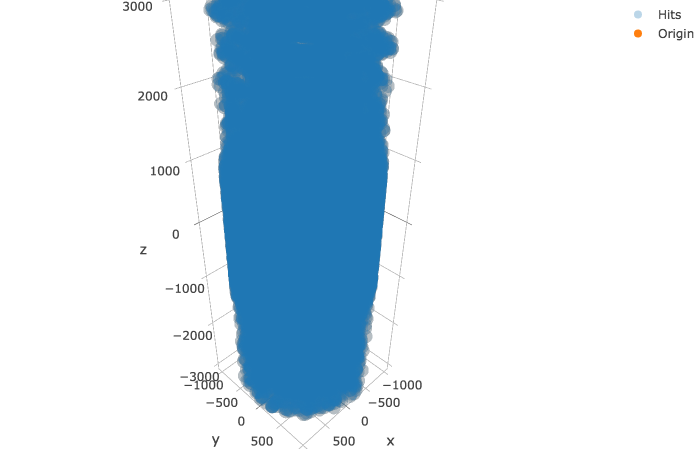In :
# Two test tracks
track1 = truth[truth.particle_id == truth.loc.particle_id]
track2 = truth[truth.particle_id == truth.loc.particle_id]

track1

Out:
hit_id particle_id tx ty tz tpx tpy tpz weight dist
hit_id
20906 20906 950260552167325696 -3.715120 32.531200 -28.693501 -0.051091 0.364180 -0.275196 0.000016 43.536171
29287 29287 950260552167325696 -10.520200 71.067299 -57.998402 -0.075847 0.359430 -0.274471 0.000013 92.331202
35612 35612 950260552167325696 -20.975201 113.817001 -90.823502 -0.099410 0.351922 -0.271879 0.000010 147.116198
42232 42232 950260552167325696 -38.887001 167.647995 -132.860992 -0.131641 0.341420 -0.270685 0.000008 217.416866
73760 73760 950260552167325696 -78.810699 252.750000 -202.261993 -0.179820 0.318319 -0.266942 0.000005 333.172030
80449 80449 950260552167325696 -132.494995 332.373993 -272.451996 -0.229098 0.284209 -0.266186 0.000005 449.730457
86907 86907 950260552167325696 -244.658997 440.040009 -386.165009 -0.291042 0.219140 -0.266104 0.000005 634.520784
92821 92821 950260552167325696 -395.536011 523.101013 -512.562012 -0.341102 0.127076 -0.265053 0.000008 832.347997
108754 108754 950260552167325696 -601.302979 561.273010 -666.289001 -0.361658 0.002130 -0.266721 0.000010 1058.552668
In :
track2

Out:
hit_id particle_id tx ty tz tpx tpy tpz weight dist
hit_id
22405 22405 468379102890426368 31.363001 -2.240470 49.250099 0.555573 -0.030916 0.827233 0.000014 58.431411
31033 31033 468379102890426368 71.755699 -3.584530 109.389999 0.555913 -0.005662 0.826708 0.000012 130.873605
38293 38293 468379102890426368 115.221001 -3.005630 174.029999 0.554995 0.020648 0.825945 0.000010 208.737528
44866 44866 468379102890426368 171.330994 0.850648 258.066010 0.550073 0.054562 0.827046 0.000008 309.762964
44872 44872 468379102890426368 173.184998 1.036430 260.854004 0.550368 0.054847 0.826769 0.000007 313.111689
76732 76732 468379102890426368 260.217987 13.868400 392.063995 0.542548 0.108607 0.823840 0.000005 470.765235
83789 83789 468379102890426368 353.845001 37.641102 535.929016 0.529077 0.164183 0.822516 0.000004 643.306328
83778 83778 468379102890426368 359.763000 39.498501 545.138000 0.528109 0.166867 0.822467 0.000004 654.343182
90693 90693 468379102890426368 488.882996 90.821198 751.671021 0.496812 0.242259 0.821913 0.000004 901.257108
97056 97056 468379102890426368 636.452026 179.432999 1008.159973 0.446866 0.323151 0.822002 0.000006 1205.675744
118379 118379 468379102890426368 744.026978 269.036011 1217.500000 0.396525 0.379051 0.822572 0.000007 1451.985795
118915 118915 468379102890426368 870.002014 412.187012 1505.500000 0.321804 0.435167 0.824130 0.000008 1786.989647
In :
# Visualize test tracks

trace1 = go.Scatter3d(x=track1.tx, y=track1.ty, z=track1.tz, mode='markers', name="Track 1")
trace2 = go.Scatter3d(x=track2.tx, y=track2.ty, z=track2.tz, mode='markers', name="Track 2")
trace3 = go.Scatter3d(x=, y=, z=, mode='markers', name="Origin")
layout = go.Layout(margin=dict(l=0, r=0, b=0, t=0))
fig = go.Figure(data=[trace1, trace2, trace3], layout=layout)
#py.iplot(fig)

Image('fig2.png')

Out: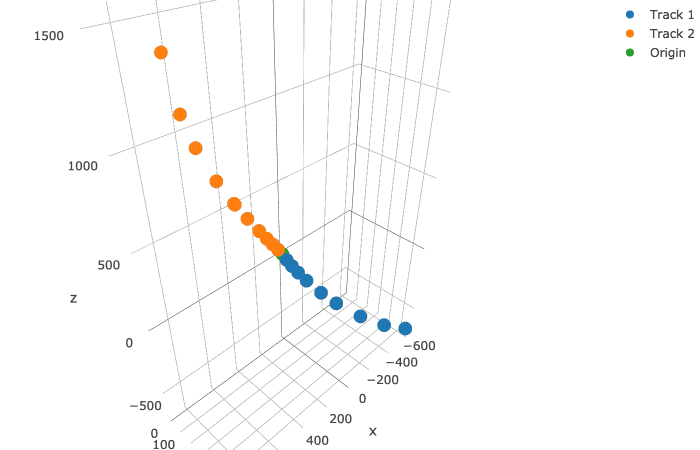Now the task is to find these two (and all of the other) tracks from the unannotated mess of points above. A logical first step is to look for the first hits of the track. One might expect these to be the ones closest to the origin point, but because of the cylindrical structure of the detector, distance isn't the way to go, as can be seen below, where all actual first hits are plottet (for orientation, both example tracks are also plottet):

In :
# True first hits

first_true = truth.drop_duplicates(subset='particle_id')

trace1 = go.Scatter3d(x=first_true.tx, y=first_true.ty, z=first_true.tz, mode='markers', opacity=0.3, name="True first hits")
trace2 = go.Scatter3d(x=track1.tx, y=track1.ty, z=track1.tz, mode='markers', opacity=1, name="Track 1")
trace3 = go.Scatter3d(x=track2.tx, y=track2.ty, z=track2.tz, mode='markers', opacity=1, name="Track 2")
trace4 = go.Scatter3d(x=, y=, z=, mode='markers', opacity=1, name="Origin")
layout = go.Layout(margin=dict(l=0, r=0, b=0, t=0))
fig = go.Figure(data=[trace1, trace2, trace3, trace4], layout=layout)
#py.iplot(fig)

Image('fig3.png')

Out: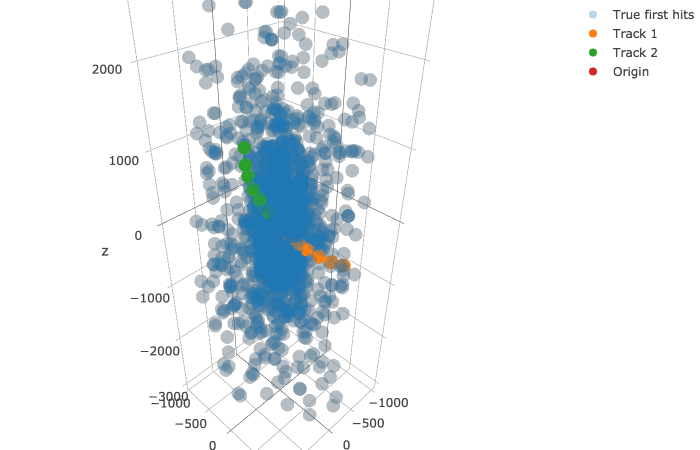It is evident that most first hits lie on the innermost cylindrical detector, as is to be expected. However, a lot of random mess can be seen everywhere, where the particle apparently was not detected by detectors closer to the origin. To predict these first hits, I used a random forest classifier.

In :
# Predict first hits

X = truth
y = truth.hit_id.isin(first_true.hit_id)
X_train, X_test, y_train, y_test = train_test_split(X, y, test_size=1/4)

model_first = RandomForestClassifier()
model_first.fit(X_train, y_train)

print("Confusion Matrix of test data:\n", confusion_matrix(y_test, model_first.predict(X_test)))

Confusion Matrix of test data:
[[27288   262]
[  501  2184]]


These results are not great, but they would probably get better if more data was used to train the classifier. Below is a plot of what the model predicts as the first hits, but remember that it was trained on 3/4 of exactly these points, so if it gets a lot correct that is not coincidence or brilliance, but just the fact that the model was fit to this data.

In :
# Predicted first hits

y_pred = model_first.predict(truth)
first_pred = truth[y_pred]

trace1 = go.Scatter3d(x=first_pred.tx, y=first_pred.ty, z=first_pred.tz, mode='markers', opacity=0.3, name="Predicted first hits")
trace2 = go.Scatter3d(x=track1.tx, y=track1.ty, z=track1.tz, mode='markers', opacity=1, name="Track 1")
trace3 = go.Scatter3d(x=track2.tx, y=track2.ty, z=track2.tz, mode='markers', opacity=1, name="Track 2")
trace4 = go.Scatter3d(x=, y=, z=, mode='markers', opacity=1, name="Origin")
layout = go.Layout(margin=dict(l=0, r=0, b=0, t=0))
fig = go.Figure(data=[trace1, trace2, trace3, trace4], layout=layout)
#py.iplot(fig)

Image('fig4.png')

Out: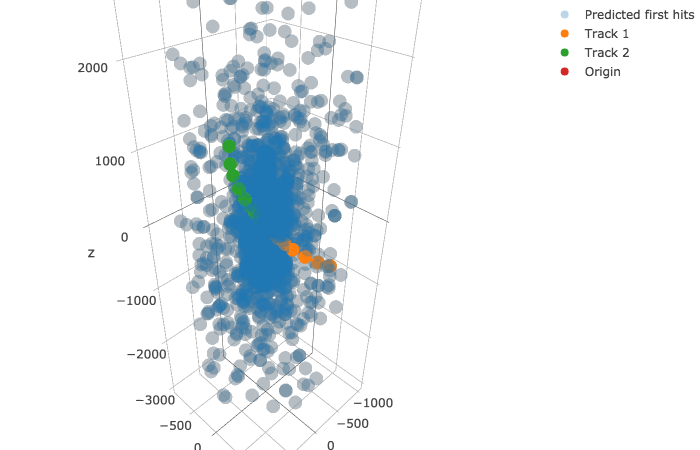In :
# Similarity

print("Total number of predicted first hits:", y_pred.sum())
print("Total errors made:", (y_pred ^ y).sum())
print("{:f}% of the prediction is correct".format((len(y) - (y_pred ^ y).sum()) / len(y)))

Total number of predicted first hits: 10184
0.992244% of the prediction is correct


With that, we can now turn to the second hits. This is where it gets more tricky, and the huge amount of data really becomes a problem. If one were to look for a second hit for every of our predicted first hits among all of the data, there would be far too many possibilities:

In :
num = len(y_pred) * (len(truth) - 1)    # We can exclude the first hit itself as second hit 🎉
print("Number of possibilities:", num)

Number of possibilities: 14626120782


Now if each of the calculations took only ten microsecond, it would still take:

In :
print(pd.Timedelta('10 us') * num)

1 days 16:37:41.207820


to predict all possible second hits for one event! Now suppose we would take the ten most likely second hits per first hit and predict the third hits for those, that would then take

In :
# This time, we can exclude two hits on our search for the third one!
num = len(y_pred) * 10 * (len(truth) - 2)
print(pd.Timedelta('10 us') * num)

16 days 22:16:39.984300


Alright, that is definetely too long. But, because we are not looking for a random point among all other ones, we can just exclude most points when looking for a second hit. For example, we know the particle physically has to continue moving away from the origin. That already excludes all hits that are closer to the origin than our first hit. Also, the second hit won't be too far away from the first hit, we can calculate the average distance of the two:

In :
l = np.array([])
for _, hits in truth.groupby("particle_id"):
if len(hits) > 1:
l = np.append(l, [np.sqrt((hits.tx.iloc - hits.tx.iloc)**2
+ (hits.ty.iloc - hits.ty.iloc)**2
+ (hits.tz.iloc - hits.tz.iloc)**2)])

print(f"The average distance between first and second hit is {l.mean():.2f} "
f"with a standard deviation of {l.std():.2f}.")

The average distance between first and second hit is 89.87 with a standard deviation of 151.94.


Now we can define a cone with the first hit being the starting point in which the second point must be. We can use efficient linear algebra libraries to rapidly calculate these cones:

In :
%%time

# First layer, I only use 5000 points because otherwise my laptop crashes.
layer = first_true.iloc[1:5001]

# Put all hits into a tensor with coordinates hit_id, and prev; prev here is the hit_id of the previous hit (namely the first hit)
x = xr.DataArray(np.resize(truth.tx.values, (len(layer), len(truth))).T, coords={'hit_id': truth.hit_id.values, 'prev': layer.hit_id.values}, dims=['hit_id', 'prev'])
y = xr.DataArray(np.resize(truth.ty.values, (len(layer), len(truth))).T, coords={'hit_id': truth.hit_id.values, 'prev': layer.hit_id.values}, dims=['hit_id', 'prev'])
z = xr.DataArray(np.resize(truth.tz.values, (len(layer), len(truth))).T, coords={'hit_id': truth.hit_id.values, 'prev': layer.hit_id.values}, dims=['hit_id', 'prev'])
ds = x.to_dataset(name='x')
ds['y'] = y
ds['z'] = z

# Put first hits in similar shape as all other hits (xarray)
X = layer[['tx', 'ty', 'tz']].to_xarray()
X = X.rename({'hit_id': 'prev', 'tx': 'x', 'ty': 'y', 'tz': 'z'})

# Calculate the angle between all first hits and possible second hits
xp = ds - X    # Elements of xp are the vectors between the first hit (x) and possible second hit (p)
xpl = (xp.x**2 + xp.y**2 + xp.z**2)**(1/2)
p = xp/xpl     # Norm all vectors

ox = X         # Elements of ox are the vectors between the origin (o) and the first hit (x)
oxl = (ox.x**2 + ox.y**2 + ox.z**2)**(1/2)
n = ox/oxl     # Norm all vectors

s = n * p      # Calculate cosine of angles between points

# Exclude all hits where the angle is > 36.9° or the distance is < 300
cones = ((((s.x + s.y + s.z) > 0.8)) & (xpl < 300)).T

CPU times: user 1min 8s, sys: 2min 54s, total: 4min 3s
Wall time: 4min 34s


To illustrate this result I will plot the cone of the first test track below:

In :
# Visualize cones
cone1 = truth[cones.sel(prev=20906).values]
trace1 = go.Scatter3d(x=cone1.tx, y=cone1.ty, z=cone1.tz, mode='markers', opacity=0.3, name="Cone")
trace2 = go.Scatter3d(x=track1.tx, y=track1.ty, z=track1.tz, mode='markers', opacity=1, name="Track")
trace3 = go.Scatter3d(x=, y=, z=, mode='markers', opacity=1, name="Origin")
layout = go.Layout(margin=dict(l=0, r=0, b=0, t=0))
fig = go.Figure(data=[trace1, trace2, trace3], layout=layout)
#py.iplot(fig)

Image('fig5.png')

Out: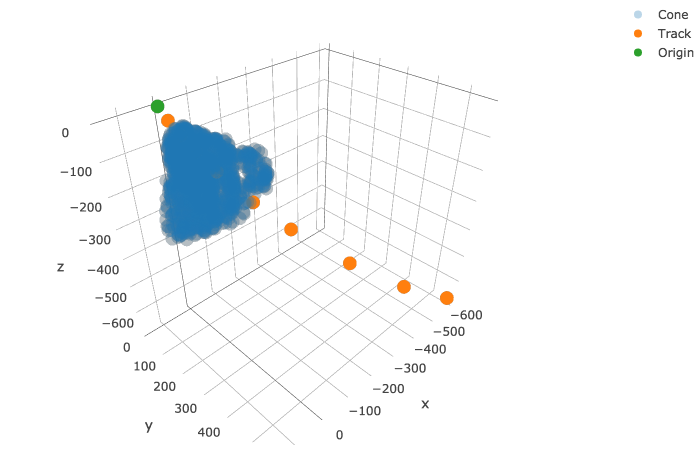Now these cones we can work with. A simple neural net can classify between "looks like a second hit" and "does not look like a second hit" among the hits inside a cone. To train the net I will use the second hit as a positive example and 10 random hits from the cone as negative example, so that the distribution isn't too skewed towards negative examples.

In :
# Process data for NN

remains = ds.where(cones)    # Hits inside cones

X = np.zeros(6)
y = np.array([])

for fhit in tqdm(ds.prev):
# Get 10 random hits from cone
remain = remains.sel(prev=fhit).dropna('hit_id')
remain = remain.sel(hit_id=np.random.choice(remain.hit_id.values, 10)).to_dataframe()

# Add the one true second hit
try:
t = truth[truth.particle_id == truth.particle_id.loc[int(fhit)]].iloc
except:
continue

remain = remain[remain.index != t.hit_id]
prev = truth.loc[np.full((len(remain),), fhit)]
y = np.concatenate((y, len(remain)*, ))
X = np.vstack([X, np.vstack([prev.tx, prev.ty, prev.tz, remain.x.values, remain.y.values, remain.z.values]).T,
[prev.tx.iloc, prev.ty.iloc, prev.tz.iloc, t.tx, t.ty, t.tz]])

X = X[1:]

100%|██████████| 5000/5000 [01:36<00:00, 51.66it/s]

In :
# Train the NN

model_second = Sequential()
model_second.compile(optimizer='rmsprop', loss='binary_crossentropy')
model_second.fit(X, y, epochs=5)

Epoch 1/5
53878/53878 [==============================] - 3s 49us/step - loss: 0.1586
Epoch 2/5
53878/53878 [==============================] - 2s 43us/step - loss: 0.1057
Epoch 3/5
53878/53878 [==============================] - 2s 42us/step - loss: 0.0973
Epoch 4/5
53878/53878 [==============================] - 2s 44us/step - loss: 0.0907
Epoch 5/5
53878/53878 [==============================] - 2s 41us/step - loss: 0.0927

Out:
<keras.callbacks.History at 0x1c33c0f320>
In :
# Test the NN on the two test tracks

cone1 = truth[cones.sel(prev=20906).values][['tx', 'ty', 'tz']].values
X1 = np.hstack([np.repeat(truth.loc[['tx', 'ty', 'tz']].values[np.newaxis], len(cone1), axis=0), cone1])
second_1 = cone1[np.argmax(model_second.predict(X1))]

cone2 = truth[cones.sel(prev=22405).values][['tx', 'ty', 'tz']].values
X2 = np.hstack([np.repeat(truth.loc[['tx', 'ty', 'tz']].values[np.newaxis], len(cone2), axis=0), cone2])
second_2 = cone2[np.argmax(model_second.predict(X2))]

trace1 = go.Scatter3d(x=, y=, z=, mode='markers', opacity=1, name="Origin")

trace2 = go.Scatter3d(x=track1.tx.iloc[2:], y=track1.ty.iloc[2:], z=track1.tz.iloc[2:], mode='markers', opacity=1, name="Track 1")
trace3 = go.Scatter3d(x=[track1.tx.iloc], y=[track1.ty.iloc], z=[track1.tz.iloc], mode='markers', opacity=1, name="First hit 1")
trace4 = go.Scatter3d(x=[track1.tx.iloc], y=[track1.ty.iloc], z=[track1.tz.iloc], mode='markers', opacity=1, name="Second hit 1 (true)")
trace5 = go.Scatter3d(x=[second_1], y=[second_1], z=[second_1], mode='markers', opacity=1, name="Second hit 1 (predicted)")

trace6 = go.Scatter3d(x=track2.tx.iloc[2:], y=track2.ty.iloc[2:], z=track2.tz.iloc[2:], mode='markers', opacity=1, name="Track 2")
trace7 = go.Scatter3d(x=[track2.tx.iloc], y=[track2.ty.iloc], z=[track2.tz.iloc], mode='markers', opacity=1, name="First 2 hit")
trace8 = go.Scatter3d(x=[track2.tx.iloc], y=[track2.ty.iloc], z=[track2.tz.iloc], mode='markers', opacity=1, name="Second 2 hit (true)")
trace9 = go.Scatter3d(x=[second_2], y=[second_2], z=[second_2], mode='markers', opacity=1, name="Second hit 2 (predicted)")

layout = go.Layout(margin=dict(l=0, r=0, b=0, t=0))
fig = go.Figure(data=[trace1, trace2, trace3, trace4, trace5, trace6, trace7, trace8, trace9], layout=layout)
#py.iplot(fig)

Image('fig6.png')

Out: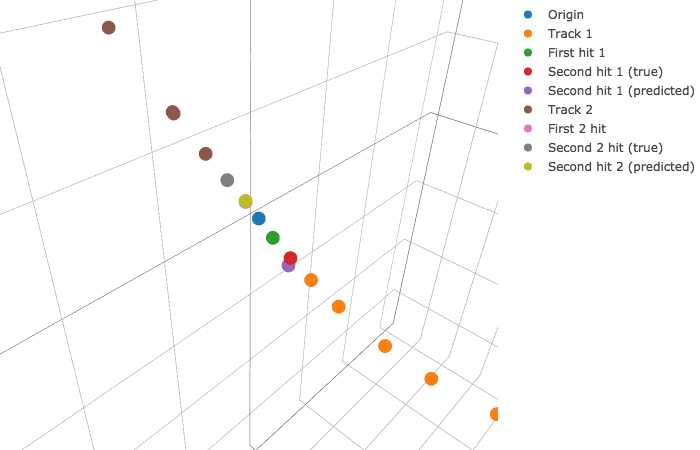The model accurately predicts the second hit of the first track, but fails to predict the second hit of the second track, though on a previous trial the same network predicted the second hit of the first track accurately.

To predict the third and subsequent hits we will use neural nets that use the previous hits as input and output the predicted position in space of the next hit. Then we can use the closest available hit as a prediction.

In :
# Process data for NN

X = np.zeros(6)
y = np.zeros(3)

for fhit in tqdm(ds.prev):
t = truth[truth.particle_id == truth.particle_id.loc[int(fhit)]]
try:
y = np.vstack([y, np.r_[t.iloc.tx, t.iloc.ty, t.iloc.tz]])
X = np.vstack([X, np.r_[t.iloc.tx, t.iloc.ty, t.iloc.tz,
t.iloc.tx, t.iloc.ty, t.iloc.tz]])
except:
continue

X = X[1:]
y = y[1:]

100%|██████████| 5000/5000 [00:14<00:00, 345.75it/s]

In :
# Train the NN

model_third = Sequential()
model_third.compile(optimizer='rmsprop', loss='mse')
model_third.fit(X, y, epochs=20)

Epoch 1/20
4837/4837 [==============================] - 0s 96us/step - loss: 2230.0851
Epoch 2/20
4837/4837 [==============================] - 0s 39us/step - loss: 838.3017
Epoch 3/20
4837/4837 [==============================] - 0s 38us/step - loss: 825.0221
Epoch 4/20
4837/4837 [==============================] - 0s 37us/step - loss: 825.1955
Epoch 5/20
4837/4837 [==============================] - 0s 38us/step - loss: 783.8190
Epoch 6/20
4837/4837 [==============================] - 0s 38us/step - loss: 784.6124
Epoch 7/20
4837/4837 [==============================] - 0s 39us/step - loss: 784.2446
Epoch 8/20
4837/4837 [==============================] - 0s 40us/step - loss: 773.4479
Epoch 9/20
4837/4837 [==============================] - 0s 38us/step - loss: 752.6868
Epoch 10/20
4837/4837 [==============================] - 0s 39us/step - loss: 754.0583
Epoch 11/20
4837/4837 [==============================] - 0s 37us/step - loss: 755.4317
Epoch 12/20
4837/4837 [==============================] - 0s 37us/step - loss: 749.5753
Epoch 13/20
4837/4837 [==============================] - 0s 38us/step - loss: 760.9674
Epoch 14/20
4837/4837 [==============================] - 0s 38us/step - loss: 755.6223
Epoch 15/20
4837/4837 [==============================] - 0s 38us/step - loss: 743.6794
Epoch 16/20
4837/4837 [==============================] - 0s 38us/step - loss: 737.1274
Epoch 17/20
4837/4837 [==============================] - 0s 38us/step - loss: 738.6237
Epoch 18/20
4837/4837 [==============================] - 0s 39us/step - loss: 734.3788
Epoch 19/20
4837/4837 [==============================] - 0s 39us/step - loss: 737.1564
Epoch 20/20
4837/4837 [==============================] - 0s 36us/step - loss: 739.3553

Out:
<keras.callbacks.History at 0x1c3143b7f0>
In :
# Test the NN on the two test tracks

X1 = np.r_[track1.tx.iloc, track1.ty.iloc, track1.tz.iloc, track1.tx.iloc, track1.ty.iloc, track1.tz.iloc]
third_1 = model_third.predict(X1[np.newaxis])    # Predicted position
third_1 = truth.iloc[np.argmin(np.sqrt(np.sum((truth[['tx', 'ty', 'tz']].values - third_1)**2, axis=1)))]    # Nearest hit

X2 = np.r_[track2.tx.iloc, track2.ty.iloc, track2.tz.iloc, track2.tx.iloc, track2.ty.iloc, track2.tz.iloc]
third_2 = model_third.predict(X2[np.newaxis])
third_2 = truth.iloc[np.argmin(np.sqrt(np.sum((truth[['tx', 'ty', 'tz']].values - third_2)**2, axis=1)))]    # Nearest hit

trace1 = go.Scatter3d(x=, y=, z=, mode='markers', opacity=1, name="Origin")

trace2 = go.Scatter3d(x=track1.tx, y=track1.ty, z=track1.tz, mode='markers', opacity=1, name="Track 1")
trace3 = go.Scatter3d(x=[track1.tx.iloc], y=[track1.ty.iloc], z=[track1.tz.iloc], mode='markers', opacity=1, name="Third hit 1 (true)")
trace4 = go.Scatter3d(x=[third_1.tx], y=[third_1.ty], z=[third_1.tz], mode='markers', opacity=1, name="Third hit 1 (predicted)")

trace5 = go.Scatter3d(x=track2.tx, y=track2.ty, z=track2.tz, mode='markers', opacity=1, name="Track 2")
trace6 = go.Scatter3d(x=[track2.tx.iloc], y=[track2.ty.iloc], z=[track2.tz.iloc], mode='markers', opacity=1, name="Third hit 2 (true)")
trace7 = go.Scatter3d(x=[third_2.tx], y=[third_2.ty], z=[third_2.tz], mode='markers', opacity=1, name="Third hit 2 (predicted)")

layout = go.Layout(margin=dict(l=0, r=0, b=0, t=0))
fig = go.Figure(data=[trace1, trace2, trace3, trace4, trace5, trace6, trace7], layout=layout)
#py.iplot(fig)

Image('fig7.png')

Out: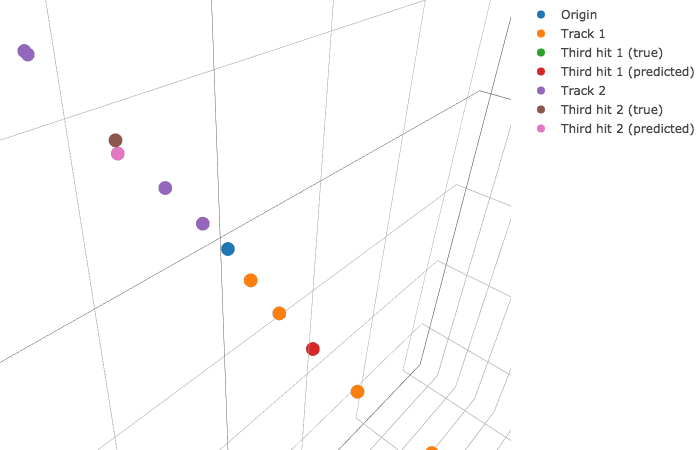The model fails to predict the third hit for the second track, but correctly predicts the third hit for the first track. Again, the model probably would get better if it were trained on a lot more data, but this algorithm is also very rudimentary and can be improved a great deal if more time was invested.

However, I have already missed the deadline on this kaggle competition and won't continue work from here on. Thank you very much for reading :)